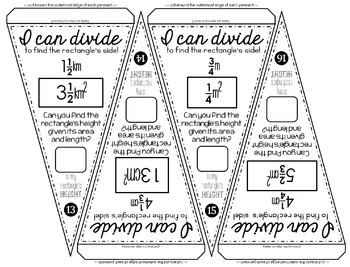# Dividing Fractions Pennant Activity

Rated 4.82 out of 5, based on 45 reviews
45 Ratings;
5th - 6th
Subjects
Resource Type
Standards
Formats Included
• PDF
Pages
8 pages
Report this resource to TPT
##### Also included in
1. This bundle includes 6 sets of fractions pennants for comparing, adding, subtracting, multiplying, dividing and converting. Each set includes math pennants for students to color, an optional student answer sheet and answer keys.Math pennants are a fun way to show off student work. Students love seei
Price \$12.50Original Price \$21.00Save \$8.50

### Description

Students divide fractions by finding missing lengths of rectangles given area and height in this activity that doubles as classroom décor. This links fraction division to the area model to make the concept more concrete. Students need to change mixed numbers to improper fractions first before dividing. The answer key gives answers in mixed number form. You may ask your students to give answers in mixed numbers or improper fractions.

Units of measure are in cm, m and km.

Once a pennant is complete, it can be hung along a string in your classroom to show the world that, "Hey, we know how to divide fractions!"

Included are 20 pennants with fraction division problems, an optional student answer sheet and an answer key. Each group of students can be given a set of pennants, crayons, scissors and either glue, tape or a stapler. Students can be in charge of cutting out the pennants. Groups can compete to see which group can complete the most pennants or your entire class can add pennants to one string as they complete each one.

You may also like:

Fraction Pennants Bundle

Dividing Fractions and Mixed Numbers Digital Math Escape Room

Total Pages
8 pages
Included
Teaching Duration
N/A
Report this resource to TPT
Reported resources will be reviewed by our team. Report this resource to let us know if this resource violates TPT’s content guidelines.

### Standards

to see state-specific standards (only available in the US).
Interpret and compute quotients of fractions, and solve word problems involving division of fractions by fractions, e.g., by using visual fraction models and equations to represent the problem. For example, create a story context for (2/3) ÷ (3/4) and use a visual fraction model to show the quotient; use the relationship between multiplication and division to explain that (2/3) ÷ (3/4) = 8/9 because 3/4 of 8/9 is 2/3. (In general, (𝘢/𝘣) ÷ (𝘤/𝘥) = 𝘢𝘥/𝘣𝘤.) How much chocolate will each person get if 3 people share 1/2 lb of chocolate equally? How many 3/4-cup servings are in 2/3 of a cup of yogurt? How wide is a rectangular strip of land with length 3/4 mi and area 1/2 square mi?